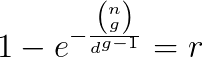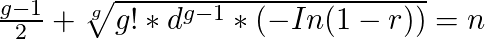Friday, 23 June 2017

More Than 2 People Sharing The Same Birthday

There are very few coding examples out there when it comes to calculating the probability of more than 2 people sharing the same birthday. To make this work I have used this Poisson approximation equation, in generalised form this equation looks like this:

n = random people
g = pairs
d = days
r = probabilityHere is the source code:
```  public static double ProbabilityOfPair(int numOfRandomInstances, int uniquePossibilities, int numOfPairs = 2)
{
if (numOfPairs < 2)
throw new Exception("Cannot have less than two pairs");

if (numOfRandomInstances < 1 || uniquePossibilities < 1)
throw new Exception("Number of random instances or unique possibilities needs to be greater than 0");

double bionomial = calcBinomialCoefficient(numOfRandomInstances, numOfPairs);
double totalPairPossibilities = Math.Pow(uniquePossibilities, numOfPairs - 1);
return 1 - Math.Exp(-(bionomial / totalPairPossibilities));
}

static double calcBinomialCoefficient(double n, double k)
{
if (k > n)
throw new Exception("n must be greater than k");

double result = 1;
for(int i = 1; i <= k; i++)
{
result *= n - (k - i);
result /= i;
}
return result;
}
```

Birthday Problem Reverse

Now what do you do if you want to reverse the birthday problem and instead get back number of random people required to reach certain probability? For example, instead of this:
`  ProbabilityOfPair(23, 365, 2) // returns 0.5000017521827107`
You would like to do something like this:
```  FindNumOfRandomInstancesRequired(365, 2, 0.50) // returns 23
```

To begin with I’ve used ProbabilityOfPair function and just did a linear search. This did not scale very well at all, so I have decided to use binary search algorithm instead. This has worked very well, however it still felt inefficient. So, I have decided to rearrange the above equation, this is what I have ended up with:

n = random people
g = pairs
d = days
r = probabilityHere is the source code:
```  public static int FindNumOfRandomInstancesRequired(int uniquePossibilities, int numOfPairs, double requiredProbability)
{
if (requiredProbability < 0.00 || requiredProbability >= 1.00)
throw new Exception("Required probability must be between 0.00 and 1.00");

double inverse = -Math.Log(1.0 - requiredProbability);
double bionomialFact = factorial(numOfPairs);
double totalPairPossibilities = Math.Pow(uniquePossibilities, numOfPairs - 1);
double precision = (numOfPairs - 1.0) / 2.0;
double numOfRandomInstancesRequired = Math.Pow(bionomialFact * totalPairPossibilities * inverse, 1.0 / numOfPairs) + precision;

return (int)Math.Round(numOfRandomInstancesRequired, 0, MidpointRounding.AwayFromZero);
}

static int factorial(int i)
{
if (i <= 1)
return 1;
return i * factorial(i - 1);
}
```

Full Source Code (licensed under the GNU licence v3.0)

```  public class BirthdayProblemPoissonApprox
{
/// <summary>
/// Generalised birthday problem probability calculation using poisson approximation.
/// </summary>
/// <example>
///  ProbabilityOfPair(23, 365), returns 0.5000017521827107
///  ProbabilityOfPair(30, 365, 3), returns 0.030015086543320635
/// </example>
/// <param name="numOfRandomInstances">Number of random instances in the group e.g. number of random people in the group</param>
/// <param name="uniquePossibilities">Number of unique possibilities that random instances can fit into e.g. number of unique days</param>
/// <param name="numOfPairs">Number of pairs</param>
/// <see cref="https://en.wikipedia.org/wiki/Birthday_problem"/>
/// <remarks>To make this scale beyond 2 pairs, I have used equations from here: https://math.stackexchange.com/questions/25876/probability-of-3-people-in-a-room-of-30-having-the-same-birthday </remarks>
/// <returns>Probability of pair</returns>
public static double ProbabilityOfPair(int numOfRandomInstances, int uniquePossibilities, int numOfPairs = 2)
{
if (numOfPairs < 2)
throw new Exception("Cannot have less than two pairs");

if (numOfRandomInstances < 1 || uniquePossibilities < 1)
throw new Exception("Number of random instances or unique possibilities needs to be greater than 0");

double bionomial = calcBinomialCoefficient(numOfRandomInstances, numOfPairs);
double totalPairPossibilities = Math.Pow(uniquePossibilities, numOfPairs - 1);
return 1 - Math.Exp(-(bionomial / totalPairPossibilities));
}
/// <summary>
/// Generalised birthday problem reversed, find number of random instances required to fit certain probability.
/// </summary>
/// <example>
/// FindNumOfRandomInstancesRequired(365, 2, 0.50), returns 23
/// FindNumOfRandomInstancesRequired(365, 3, 0.03), returns 30
/// </example>
/// <param name="uniquePossibilities">Number of unique possibilities that random instances can fit into e.g. number of unique days</param>
/// <param name="numOfPairs">Number of pairs</param>
/// <param name="requiredProbability">Probability that must be reached</param>
/// <returns>Number of random instances required</returns>
public static int FindNumOfRandomInstancesRequired(int uniquePossibilities, int numOfPairs, double requiredProbability)
{
if (requiredProbability < 0.00 || requiredProbability >= 1.00)
throw new Exception("Required probability must be between 0.00 and 1.00");

double inverse = -Math.Log(1.0 - requiredProbability);
double bionomialFact = factorial(numOfPairs);
double totalPairPossibilities = Math.Pow(uniquePossibilities, numOfPairs - 1);
double precision = (numOfPairs - 1.0) / 2.0;
double numOfRandomInstancesRequired = Math.Pow(bionomialFact * totalPairPossibilities * inverse, 1.0 / numOfPairs) + precision;

return (int)Math.Round(numOfRandomInstancesRequired, 0, MidpointRounding.AwayFromZero);
}

/// <summary>
/// Calculates efficiently number of possible combinations, "n choose k".
/// </summary>
/// <example>For example, suppose you have a deck of 5 cards (n = 5) and you want to know how many different hands of 3 cards you can make (k = 3). If you number the cards 1 through 5, then you will get 10 possible combinations.</example>
/// <param name="n">Total set of items</param>
/// <param name="k">Number of items to pick from n</param>
/// <see cref="http://www.vb-helper.com/howto_calculate_n_choose_k.html"/>
/// <returns></returns>
static double calcBinomialCoefficient(double n, double k)
{
if (k > n)
throw new Exception("n must be greater than k");

double result = 1;
for(int i = 1; i <= k; i++)
{
result *= n - (k - i);
result /= i;
}
return result;
}

static int factorial(int i)
{
if (i <= 1)
return 1;
return i * factorial(i - 1);
}
}
```